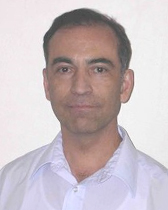# DEPARTMENT OF MATHEMATICS

## Miguel A. Lerma

### Technical Support Spec Sr.Research Area: Math Problem Solving Techniques - My interests focus on techniques for solving challenging elementary Math problems, such as proof by induction, inequalities, generating functions, counting, pigeonhole principle, telescoping, and various techniques from number theory, algebra, and calculus. A typical problem it this area would be the following:

Find a real number $c$ and a positive number $L$ for which

$\lim_{r\to\infty} \frac{r^c \int_0^{\pi/2} x^r \sin x \,dx}{\int_0^{\pi/2} x^r \cos x \,dx} = L.$

Other areas of interest: Analytic Number Theory, Computability, Logic, Foundations, Math Problem Solving, Putnam Competition Training.

More recentlly I have become interested in Artificial Intelligence, Neural Networks and Deep Learning.